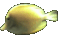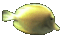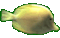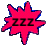Math Help Fun    Integer a/b Division    (a is -12 to 12)   (b is -12 to 12)

- The positive and negative sign rules for integer division are the same as for integer multiplication.

- When dividing 2 integers having the same sign, the result is positive. Example (-20)/(-5)= 4.
- When dividing 2 integers having different sign, the result is negative. Example (-18)/(+3)= -6.

- Your Game Score is reduced by the number of fish hits.

- To slow the game speed repeat tap/click on the word Slider.
- To increase the game speed repeat tap/click on the word Math.
- Speed can also be adjusted with a keyboard's - and + keys.

- Refresh/Reload the web page to restart the game.